# R vs Python for Machine Learning

There are so many things to learn before to choose which language is good for Machine Learning. We will discuss each and everything about R as well as Python and the situation or problem in which situation we have to use which language. Let’s start

Python and R are the two most Commonly used Programming Languages for Machine Learning and because of the popularity of both the languages Novice or you can say fresher are getting confused, whether they should choose R or Python language to commence their career in the Machine learning domain. Don’t worry guys through this article we will discuss R vs Python for Machine Learning. So, without exaggerating this article let’s get started.

We will start it from the very Basics things or definitions.

## R vs Python for Machine Learning

### Introduction

R is a programming language made by statisticians and data miners for statistical analysis and graphics supported by R foundation for statistical computing. R also provides high-quality graphics and it also has some popular libraries which help in analytical parts such as R Markdown and shiny.

On the other hand, Python is a simple, easy, fully-fledged and object-oriented high programming language which is used for web development or Software Development made by the very good programmers and the developers’ for the use of general purpose programming. Python is far-flung used in GUI based application’s such of them are games, graphics design, Web applications.

So, guys we can say that R programming language functionality is developed by statisticians’ mind, by thereby give us an advantage in a specific field. While python is often praised for being a general-purpose language with an easy-to-understand.

### Speed

Let us start from the very first factor, that is the speed of the language.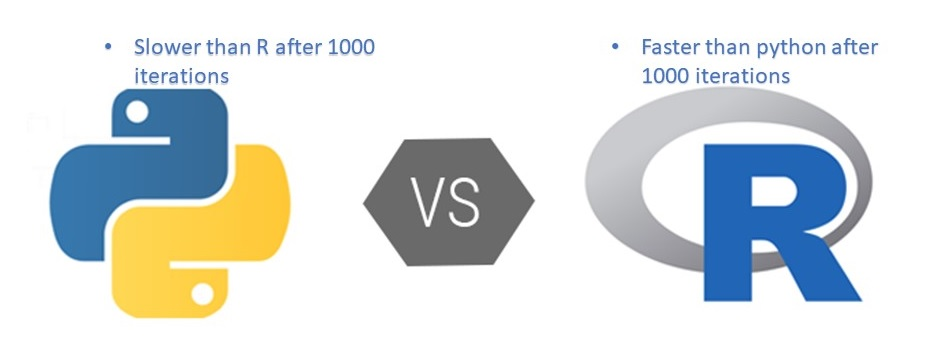#### Advertising (adsbygoogle = window.adsbygoogle || []).push({});

When it comes to the speed, python is faster than R only till 1000 iterations but after the 1000 iterations, R starts using the lapply function which increases its speed, in that situation R becomes faster than python. So, both have their own advantages with their limits. let move to the next point i.e code and syntax.

### Code and Syntax

In this point, we will discuss the data variables declarations, Data handling capacity with the scatterplot visualization and the clusPlot graphics.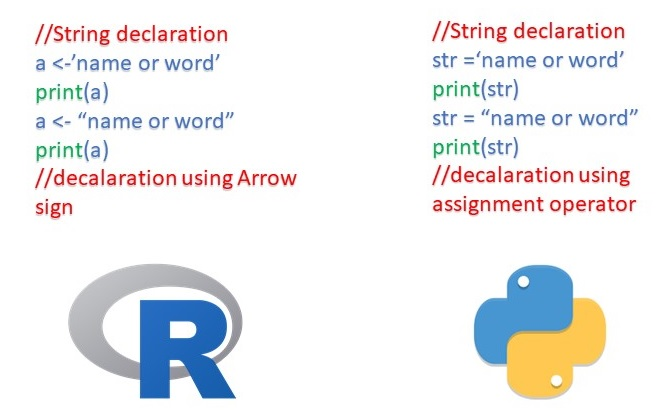Starting with variable declaration. Let’s take the case of string here. As R uses the similar implementation to that of the S programming language, which uses arrow sign in order to initialize the variables which were also present in case of S programming. These arrows can be used from right to left or left to right indicating whom to assign the variables whereas, python uses an assignment operator to initialize the variables.

So, Basically, R developers thought that it would be better to tell the direction of the assignment rather than just using an assignment operator, which could actually confuse any new programmer about which variable is assigned. next thing data handling capability, here we will discuss the case of Scatterplots’, by which you will see the visualizations in R and Python.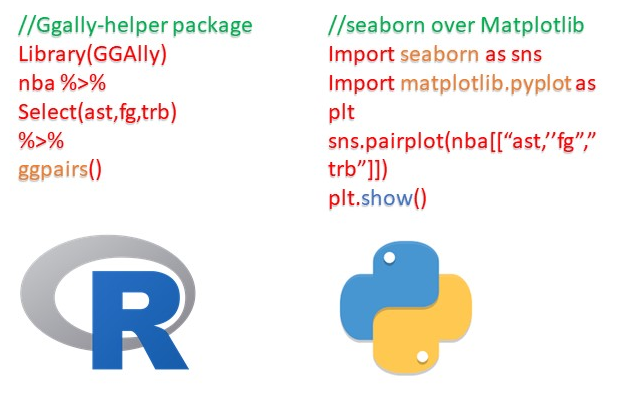These are the piece of codes in R and Python and after running  these codes, you will get the very similar plot results in both the cases, if you check the code here, then this shows that how R data science ecosystem has many smaller packages like GGally, which basically is a package that helps ggplot2 and also it is the most-used R plotting package whereas, In Python, matplotlib  is the  primary plotting package, and seaborn is widely used layer over the matplotlib. So, these are plots result we were talking about. Graph results of R and Python are both similar, but the only difference is their visualization. So, based on the graph results we can conclude that R has Many packages supporting different method of doing things whereas there is usually one way to do something in python. Moving to the next thing that is graphics.

So, guys here we will discuss the case plots, we already discussed that R was basically built for statistically analysis, so it has many specific libraries for plotting as well. This is the reasons R come up with beautiful graphs and charts whereas python’s main agenda, not for statistical analysis. So, in the early stages of the python packages for data analysis was an issue, but it has improved a lot.

### Deep Learning

As you all know almost the majority of the companies are working on Artificial Intelligence (AI), and Deep Learning is the main part of artificial intelligence. So, when it comes to Deep Learning, Python is more versatile then R as it provides more features to deep learning whereas R is new to Deep Learning.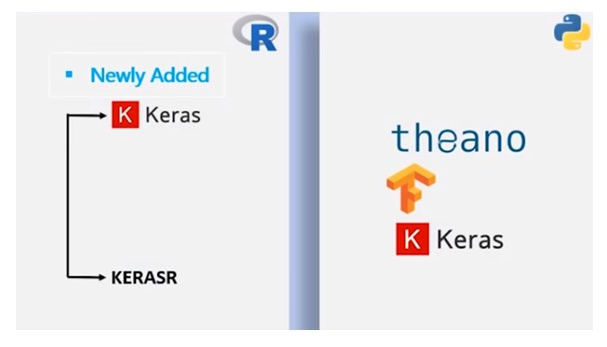R has newly added APIs like Keras and KerasR which are written in Python. So, guys somewhere in your mind, this question might be floating why Keras? Actually, Keras in Python has the capabilities to run over pythons’ strong APIs like tensorflow or Theano or Microsoft’s CNTK we can say that python has the greater advantage here. Till now we learn both are useful in their own areas or terms.

### Percentage Switching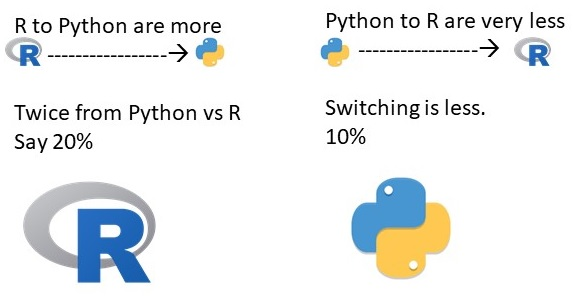In the past years of Research, the percentage of switching people R to Python are more as compared to Python to R. Let’s say if 10% people are switching from Python to R then, 20% are switching from R to Python which is double as compared to the before scenario. Next point this about trend community support and jobs.

### TrendsSo, guys lets talk about trend according to the google in last 5 years. The R was more in use but after that, we can see Python is in trend because of its popularity it has overall good support of general purpose programming. If we talk about community support: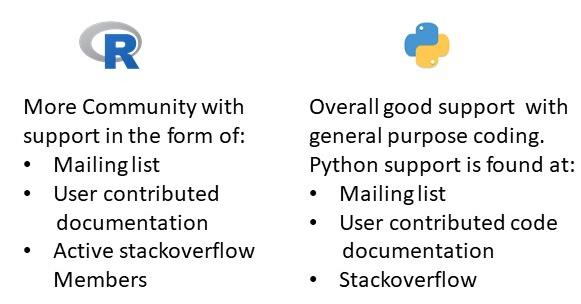Python and R support are quite similar to each other because python supports Mailing list, User-contributed code documentation, and Stack-overflow. So, basically it has more adoption from developers and programmers whereas R language support as also found at Mailing list, User-contributed documentation, and Active Stack-overflow members So, basically R has more adoption for researchers, DataScientist and Statisticians.

Job Trend

Now, lets talk about the job trend.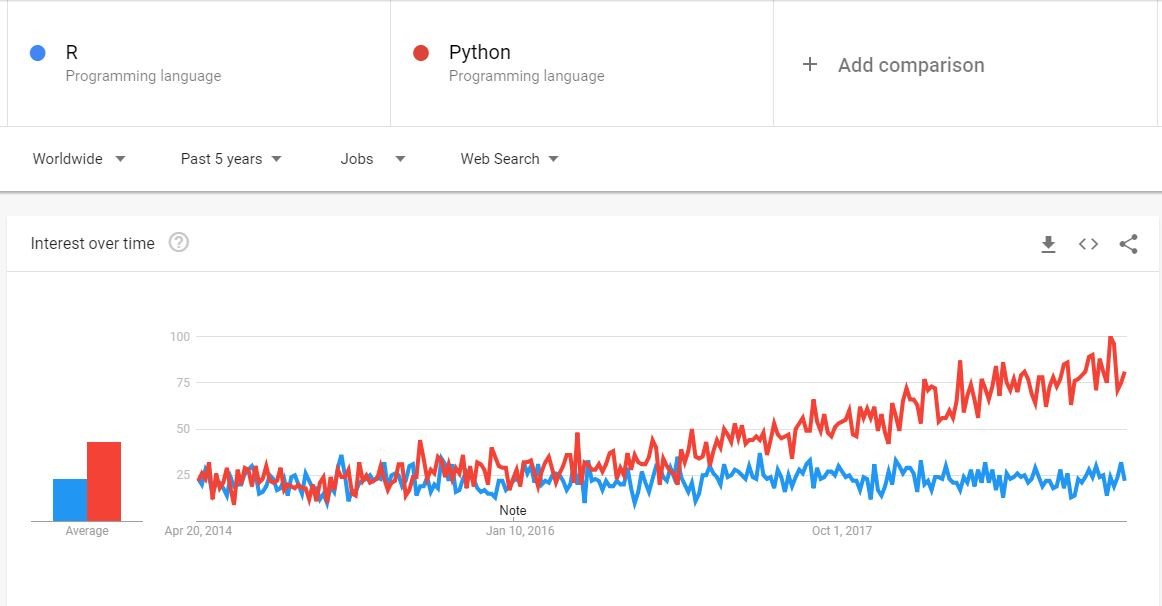This is the google graph of the job trend of Python and R. So, guys this Job Posting of R and Python in past 5 years worldwide whereas Python is asked more in comparison to R. How it is possible? because of its popularity and easy to understand feature. Since python is a very versatile programming language which can be used for majority of the purpose such as web-development, game development, artificial intelligence, data science, statistical analysis, etc, whereas R language used among statisticians and Data miners for developing statistical software and Data analysis which clear us that’s why more job for python than R.

In the end, I would like to say both the programming languages are important with their uses. But as we discussed in the previous section python is booming over the years.

The post R vs Python for Machine Learning appeared first on The Crazy Programmer.